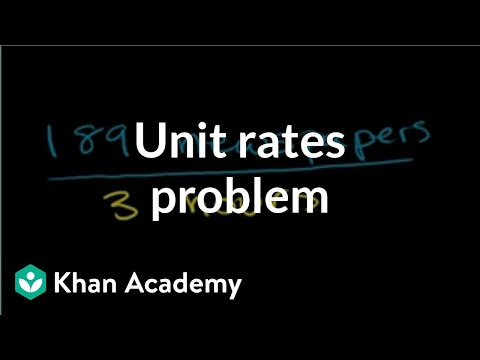# Solve Problems Involving Rates and Ratios

##By Khan Academy

Watch this video as the instructor clearly explains how to solve a word problem involving finding unit rates. When rates are expressed as a quantity of 1, such as 2 feet per second or 5 miles per hour, they are called unit rates. See how you can apply this process to help you solve similar problems.# Solve Problems Involving Rates and Ratios

##By Khan Academy

Watch this video as the instructor clearly explains how to solve a word problem involving finding unit rates. When rates are expressed as a quantity of 1 such as 2 feet per second or 5 miles per hour they are called unit rates. See how you can apply this process to help you solve similar problems.# How to use Ratios and Proportions to Solve Real World Problems

##By mrtedmartin

Instructor uses a Power Point presentation to demonstrate how to use ratios and proportions to solve problems real world problems. Unit rates are discussed and definitions are given. Solving unit rate problems are modeled and a calculator is used for computation.# Comparing rates

##By Khan Academy

Finding a unit rate is a skill often required in real life. How fast is that plane flying? How many lawns can you mow in an afternoon? You see, with our knowledge of ratios and fractions, we can now solve unit rates problems like this.# Speed translation | Ratios, proportions, units, and rates | Pre-Algebra | Khan Academy

##By Khan Academy

This video takes us through some more advanced unit conversion problems. Here, we can see how to make multiple conversions in the same problem concerning speed. Take particular note of how the units change in each step. More importantly, note how, step by step, our units slowly change into the units we want for our final answer. If you are having trouble understanding why this happens, go to the key concept described at 2:20 in the video. Note that this only occurs when multiplying two quantities, not when adding them!# Finding average speed or rate | Ratios, proportions, units, and rates | Pre-Algebra | Khan Academy

##By Khan Academy

Using the formula for finding distance we can determine Usian Bolt's average speed, or rate, when he broke the world record in 2009 in the 100m. Watch.# Using Tables to Explore Ratios and Rates

##By TeacherTube Math

YouTube presents 64 Using Tables to Explore Ratios and Rates an educational video resource on math.# Basic rate problems

##By Khan Academy

Finding a unit rate is a skill often required in real life. How fast is that plane flying? How many lawns can you mow in an afternoon? You see, with our knowledge of ratios and fractions, we can now solve unit rates problems like this.# Basic rate problems

##By Khan Academy

Finding a unit rate is a skill often required in real life. How fast is that plane flying? How many lawns can you mow in an afternoon? You see, with our knowledge of ratios and fractions, we can now solve unit rates problems like this.# Basic rate problems

##By Khan Academy

Finding a unit rate is a skill often required in real life. How fast is that plane flying? How many lawns can you mow in an afternoon? You see, with our knowledge of ratios and fractions, we can now solve unit rates problems like this.# Basic rate problems

##By Khan Academy

Finding a unit rate is a skill often required in real life. How fast is that plane flying? How many lawns can you mow in an afternoon? You see, with our knowledge of ratios and fractions, we can now solve unit rates problems like this.# Basic rate problems

##By Khan Academy

Finding a unit rate is a skill often required in real life. How fast is that plane flying? How many lawns can you mow in an afternoon? You see, with our knowledge of ratios and fractions, we can now solve unit rates problems like this.# Basic rate problems

##By Khan Academy

Finding a unit rate is a skill often required in real life. How fast is that plane flying? How many lawns can you mow in an afternoon? You see, with our knowledge of ratios and fractions, we can now solve unit rates problems like this.# Rates and ratios

##By MathPlanetVideos

Express the ratio as a fraction in simplest form and as a decimal# YouTube: Average Rate of Change

##By dhill262

General idea behind average rate of change# Given Linear Function, Find the Rate of Change and Initial Value

##By Mathispower4u

Video showing how to find rate of change of a linear function.# Average rate of change (example 2)

##By Khan Academy

Khan Academy presents Average Rate of Change Example 2), an educational video resource on math.# How to operate with matrices

##By MathPlanetVideos

If possible then perform the indicated operation: ⎡⎣⎢21−4031⎤⎦⎥⋅[602−234]# Algebra Help: Distance, Rate, and Time

##By MathTV

For high school students. Algebra Word Problem: Distance Rate and Time using two jets. Seasoned math instructor demonstates on white board. Uses colored markers for clarification.# Trig Ratios - Part 1

##By Papapodcasts

This is a video lecture course on Basics of Trigonometry and Geometry..